# Solved 2017 Question Paper ICSE Class 10 Physics

## Section I (40 Marks)

#### Question 1(a)

A brass ball is hanging from a stiff cotton thread. Draw a neat labelled diagram showing the forces acting on the brass ball and the cotton thread. 

In the figure below, the force on the ball is the weight W acting vertically downwards and the force on the thread is the tension T acting upwards.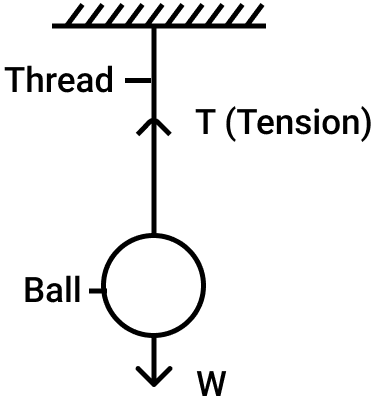#### Question 1(b)

The distance between two bodies is doubled. How is the magnitude of gravitational force between them affected? 

The gravitational force becomes one-fourth since it is inversely proportional to the square of the distance of separation (i.e., F ∝ $\dfrac{1}{d^2}$ where F is the force and d is the distance between centers)

#### Question 1(c)

Why is a jack screw provided with a long arm? 

When the effort arm is longer than the load arm then the machine acts as a force multiplier i.e., less effort is needed to overcome a large load.

For the same reason, jack screw is provided with a long arm so that a less force ( or effort) may provide the sufficient moment of force to rotate it.

#### Question 1(d)

If the power of a motor be 100 kW, at what speed can it raise a load of 50,000 N? 

Given, power P = 100 kW = 100 x 103 W, force F = 50,000 N

Power P = Force (F) x Speed (v)

Substituting the values we get,

$100 \times 10^3 = 50,000 \times v \\[0.5em] \Rightarrow v = \dfrac{100 \times 10^3 }{50,000} \\[0.5em] \Rightarrow v = 2 m s^{-1}$

#### Question 1(e)

Which class of lever will always have M.A. > 1 and why? 

From relation,

M.A. = $\dfrac{\text{Effort arm}}{\text{Load arm}}$

When effort arm > load arm then M.A. > 1.

Hence, we can say that class II lever always has M.A. > 1 because it has the effort arm always longer than the load arm.

#### Question 2(a)

Define heat capacity and state it's S.I. unit. 

Heat capacity of a body is the amount of heat energy required to raise it's temperature by 1 K.

It's S.I. unit is J K-1 (joule per kelvin).

#### Question 2(b)

Why is the base of a cooking pan generally made thick? 

By making base of a cooking pan thick, it's heat capacity becomes large due to which it gets heated slowly and it imparts sufficient heat energy at a slow rate to the food for it's proper cooking and after cooking it keeps the food warm for a long time.

#### Question 2(c)

A solid of mass 50 g at 150°C is placed in 100 g of water at 11°C, when the final temperature recorded is 20°C. Find the specific heat capacity of the solid. (Specific heat capacity of water = 4.2 J/g°C). 

Given,

Mass of solid m1 = 50 g,

temperature of solid t1 = 150°C,

mass of water m2 = 100 g

temperature of water t2 = 11°C ,

temperature of mixture t = 20°C

Let specific heat capacity of the solid be c1

Heat lost by the solid Q1 = m1 c1 (t1 - t)

= 50 x c1 x (150 - 20) = 6500 c1 J

Heat gained by water = m2 c2 (t - t2 )

= 100 x 4.2 x (20 - 11) = 3780 J

If there is no loss of heat, by the principle of calorimetry

Heat lost by the solid = Heat gained by the water

6500 c1 = 3780

c1 = $\dfrac{3780}{6500}$ = 0.582 Jg-1° C-1

#### Question 2(d)

How is the refractive index of a material related to? 

(i) real and apparent depth?

(ii) velocity of light in vacuum or air and the velocity of light in a given medium?

(i) Refractive index (μ) = $\dfrac{\text{Real depth}}{\text{Apparent depth}}$

(ii) Refractive index (μ) = $\dfrac{\text{Speed of light in vacuum or air}}{\text{\text{Speed of light in given medium }}}$

#### Question 2(e)

State the conditions required for total internal reflection of light to take place. 

The conditions required for total internal reflection are:

1. The light must travel from a denser medium to a rarer medium.
2. The angle of incidence of light in the denser medium must be greater than the critical angle for that pair of media.

#### Question 3(a)

Draw a ray diagram to show the refraction of a monochromatic ray through a prism when it suffers minimum deviation. 

The ray diagram below shows the refraction of a monochromatic ray of light through a prism in the position of minimum deviation. The refracted ray QR is parallel to the base BC of the prism or angle of incidence (i) = angle of emergence (e).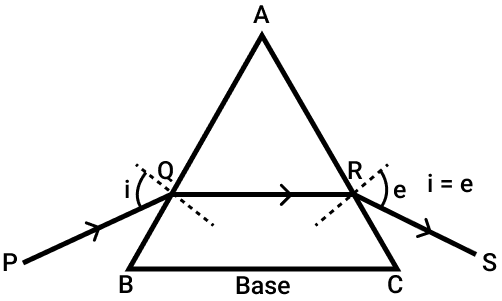#### Question 3(b)

The human ear can detect continuous sounds in the frequency range from 20 Hz to 20,000 Hz. Assuming that the speed of sound in air is 330 ms-1 for all frequencies, calculate the wavelengths corresponding to the given extreme frequencies of the audible range. 

Given,

V = 330 m s-1

fmin = 20 Hz,

fmax = 20,000 Hz

From relation, V = fλ

λmin = $\dfrac{V}{f_{max}}$ = $\dfrac{330}{20,000}$ = 1.65 x 10-2 m or 16.5 mm

and

λmax = $\dfrac{V}{f_{min}}$ = $\dfrac{330}{20}$ = 16.5 m

Thus, audible range of wavelength is from 16.5 mm to 16.5 m.

#### Question 3(c)

An enemy plane is at a distance of 300 km from a radar. In how much time the radar will be able to detect the plane? Take velocity of radio waves as 3 x 108 m s-1. 

Given,

Distance d = 300 km,

Velocity V = 3 x 108 m s-1

If it takes time t for the signal to go up to the enemy plane and come back, then

$V = \dfrac{2d}{t} \\[0.5em] \Rightarrow t = \dfrac{2d}{V} \\[0.5em] = \dfrac{2 \times (300 \times 10^3 m)}{3 \times 10^8 m s ^{-1}} \\[0.5em] = 2 \times 10^{-3} \text{ s}$

Hence, the radar will detect the enemy plane in 2 x 10-3 s

#### Question 3(d)

How is the frequency of a stretched string related to: 

(i) it's length?

(ii) it's tension?

(i) Frequency is inversely proportional to the length of the string i.e., f ∝ $\dfrac{1}{l}$

(ii) Frequency is directly proportional to the square root of the tension i.e., f ∝ √T

#### Question 3(e)

Define specific resistance and state it's S.I. unit. 

Specific resistance of a material is the resistance of a wire of that material of unit length and unit area of cross section.

The S.I. unit of specific resistance is ohm × metre or (Ω m)

#### Question 4(a)

An electric bulb of resistance 500 Ω, draws a current of 0.4 A. Calculate the power of the bulb and the potential difference at it's end. 

Given,

R = 500 Ω,

I = 0.4 A

Power of bulb P = I2 R
= (0.4)2 x 500 = 80 W

Potential difference at the ends of the bulb V = IR = 0.4 x 500 = 200 V

#### Question 4(b)

State two causes of energy loss in a transformer. 

The two causes of energy loss in a transformer are :

1. Heating loss in the wire of primary and secondary coils.
2. Hysteresis loss in the core of the transformer due to it's magnetization and demagnetization in each cycle.

#### Question 4(c)

State two characteristics of a good thermion emitter. 

The two characteristics of a good thermion emitter are :

1. It's melting point must be high.
2. It's work function must be low.

#### Question 4(d)

State two factors upon which the rate of emission of thermions depends. 

The two factors upon which the rate of emission of thermions depends are :

1. The temperature of the emitter.
2. The surface area of the emitter.

#### Question 4(e)

When does the nucleus of an atom tend to be radioactive? 

The nucleus of an atom tends to be radioactive when the number of neutrons inside it becomes more than the number of protons in it.

## Section II (40 Marks)

#### Question 5(a)

A uniform half metre rule balances horizontally on a knife edge at 29 cm mark when a weight of 20 gf is suspended from one end. 

(i) Draw a diagram of the arrangement.

(ii) What is the weight of the half metre rule?

(i) Diagram of the arrangement is shown below: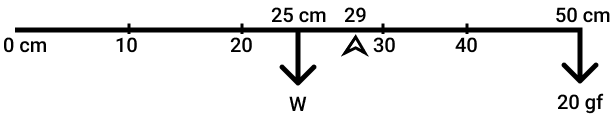(ii) If W gf is the weight of half meter rule, by the principle of moments,

$W \times (29 - 25) = 20 \times (50 - 29) \\[0.5em] \Rightarrow W \times 4 = 20 \times 21 \\[0.5em] \Rightarrow W = \dfrac{20 \times 21}{4} \\[0.5em] \Rightarrow W = 105 \text{ gf}$

#### Question 5(b)

(i) A boy uses a single fixed pulley to lift a load of 50 kgf to some height. Another boy uses a single movable pulley to lift the same load to the same height. Compare the effort applied by them. Give a reason to support your answer. 

(ii) How does uniform circular motion differs from uniform linear motion?

(iii) Name the process used for producing electricity using nuclear energy.

(i) From relation,

$\text{M.A.} = \dfrac{\text{L}}{\text{E}}$

we can say that M.A. and effort are inversely related.

Therefore, we get,

$\dfrac{\text{Single Fixed Pulley Effort}}{\text{Single Movable Pulley Effort}} = \dfrac{2}{1}$

The reason is that the mechanical advantage of a single movable pulley is 2 while that of a single fixed pulley is 1.

(ii) The difference between uniform circular motion and uniform linear motion is as follows:

Uniform circular motionUniform linear motion
In a uniform circular motion the velocity is variable (although the speed is uniform), so it is an accelerated motion.In uniform linear motion the speed and velocity are constant and acceleration is zero i.e., the uniform linear motion is an unaccelerated motion.

(ii) Nuclear fission

#### Question 5(c)

A pulley system with V.R. = 4 is used to lift a load of 175 kgf through a vertical height of 15 m. The effort required is 50 kgf in the downward direction. (g = 10 N kg-1) 

Calculate:

(i) Distance moved by the effort.

(ii) Work done by the effort.

(iii) M.A. of the pulley system.

(iv) Efficiency of the pulley system.

Given,

V.R. = 4

L = 175 kgf

dL = 15 m

E = 50 kgf

(i) V.R. = $\dfrac{d_E}{d_L}$

Substituting the values we get,

$4 = \dfrac{d_E}{15} \\[0.5em] \Rightarrow d_E = 15 \times 4 \\[0.5em] \Rightarrow d_E = 60 \text{ m}$

(ii) Work done by the effort = E x dE
= 50 x 10 x 60
= 3 x 104

(iii) M.A. = $\dfrac{L}{E}$

Substituting the values we get,

$\text {M.A.} = \dfrac{175}{50} = 3.5$

(iv) Efficiency η = $\dfrac{M.A.}{V.R.}$

Substituting the values we get,

$η = \dfrac{3.5}{4} = 0.875 \\[0.5em] \therefore η = 87.5 %$

#### Question 6(a)

(i) How is the transference of heat energy by radiation prevented in a calorimeter? 

(ii) You have a choice of three metals A, B and C, of specific heat capacities 900 Jkg-1°C-1, 380 Jkg-1°C-1 and 460 Jkg-1°C-1 respectively, to make a calorimeter. Which material will you select? Justify your answer.

(i) To prevent heat transference by radiation, the outer and inner surfaces of calorimeter are polished.

(ii) For calorimeter, metal B will be used.
The reason is, it's specific heat capacity is lowest, so it will take least heat from its contents to acquire the temperature of contents.

#### Question 6(b)

Calculate the mass of ice needed to cool 150 g of water contained in a calorimeter of mass 50 g at 32°C such that the final temperature is 5°C. 

Specific heat capacity of calorimeter = 0.4 J g-1 °C-1

Specific heat capacity of water = 4.2 Jg-1°C-1

Latent heat capacity of ice = 330 Jg-1

Given,

Mass of water m1 = 150 g

Mass of calorimeter m2 = 50 g

Temperature of water t1 = 32°C

Final temperature t = 5°C

Let m be the mass of ice added

Heat given by calorimeter Q1 = m2 c2 x (t1 - t)

= 50 x 0.4 x (32 - 5) = 540 J

Heat given by water Q2 = m1 c1 x (t1 - t)

= 150 x 4.2 x (32 - 5) = 17010 J

Heat taken by ice to melt at 0°C = m L

= m x 330 Jg-1

Heat energy taken by the melted ice to to raise it's temperature from 0°C to 5 °C

= m x 4.2 x (5 - 0) = 21m J

Total heat taken by ice = 330m + 21m = 351 m J

By principle of calorimeter, if there is no loss of heat.

351 m = 540 + 17010

m = $\dfrac{17550}{351}$ = 50 g

#### Question 6(c)

(i) Name the radiations which are absorbed by greenhouse gases in the earth’s atmosphere. 

(ii) A radiation X is focused by a particular device on the bulb of a thermometer and mercury in the thermometer shows a rapid increase. Name the radiation X.

(iii) Name two factors on which the heat energy liberated by a body depends.

(i) The green house gases in the earth's atmosphere absorb the low energy infrared radiations.

(iii) The two factors are :

1. Material of the body
2. Temperature of the body.

#### Question 7(a)

A lens forms an upright and diminished image of an object when the object is placed at the focal point of the given lens. 

(i) Name the lens.

(ii) Draw a ray diagram to show the image formation.

(i) The lens is concave

(ii) The ray diagram is shown below :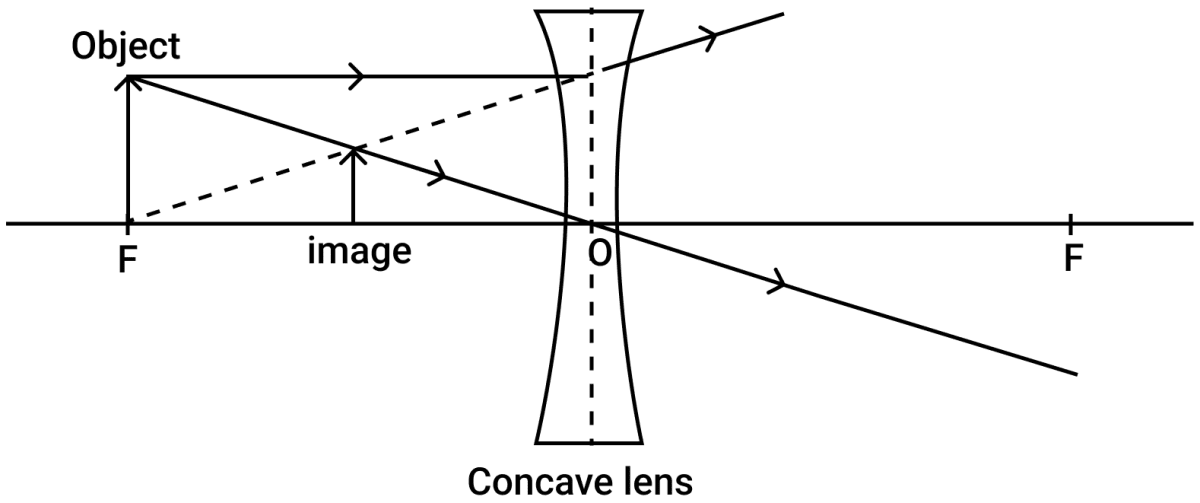#### Question 7(b)

A ray of light travels from water to air as shown in the diagram given below: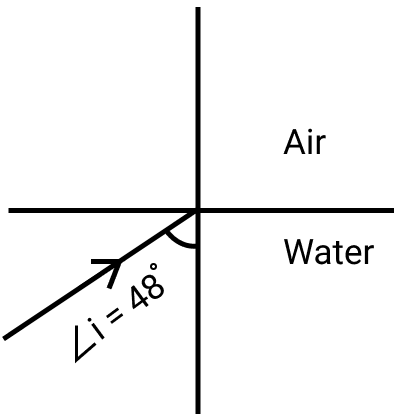(i) Copy the diagram and complete the path of the ray. Given the critical angle for water is 48°.

(ii) State the condition so that total internal reflection occurs in the above diagram.

(i) Below diagram shows the path of the ray AO after reflection as OB: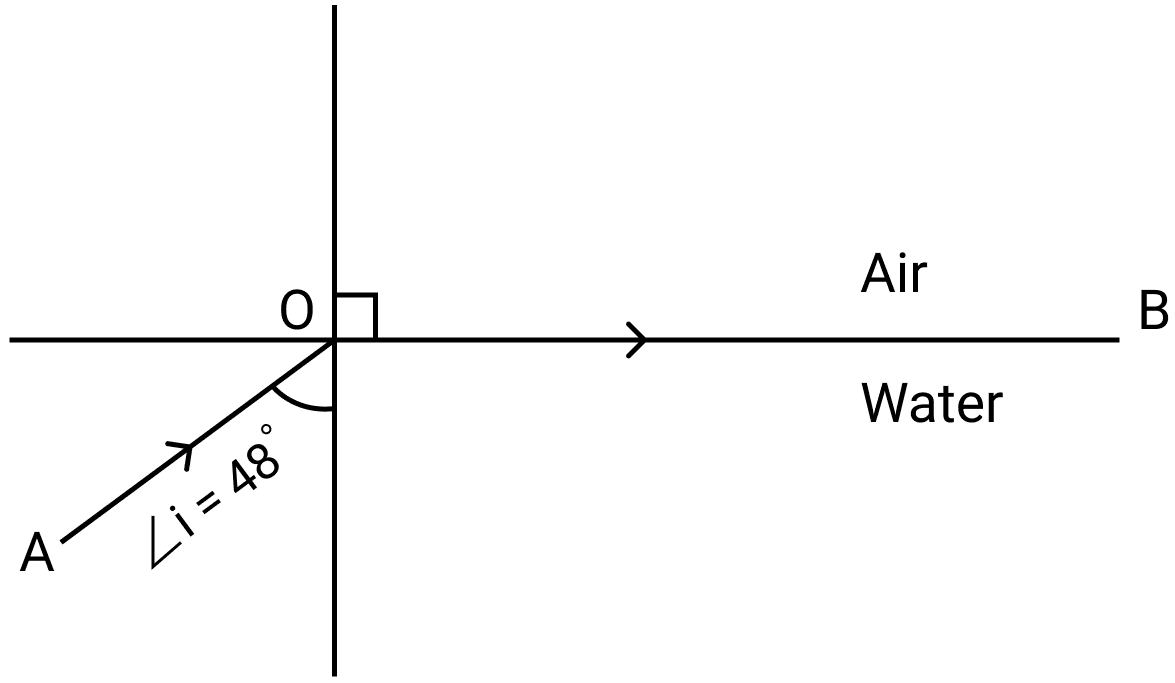(ii) For total internal reflection to occur, the angle of incidence i of incident ray AO must be more than 48° (i.e., angle i > 48 °)

#### Question 7(c)

The diagram below shows a point source P inside a water container. Four rays A, B, C, D starting from the source P are shown up to the water surface.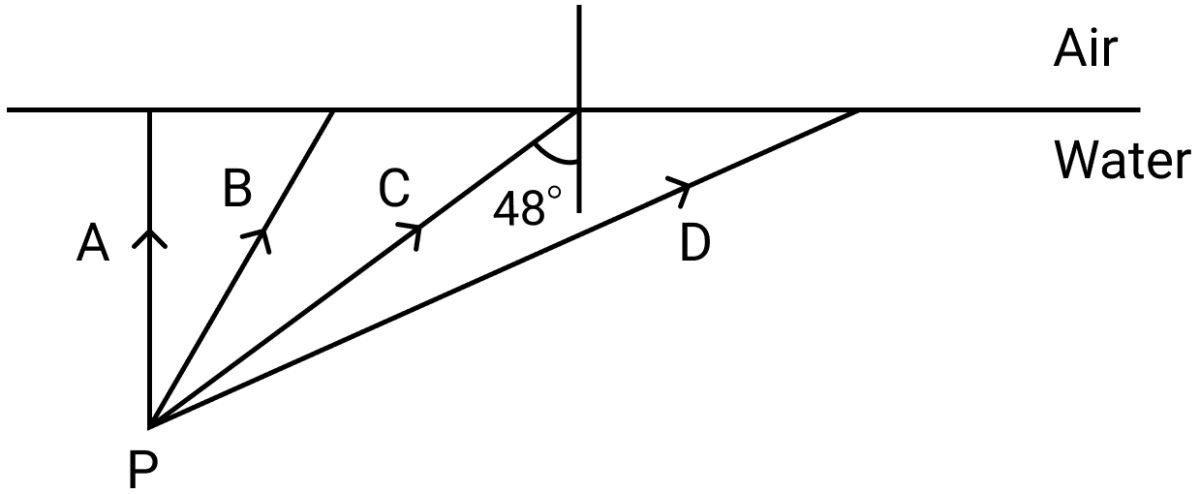(i) Show in the diagram the path of these rays after striking the water surface. The critical angle for water air surface is 48°.

(ii) Name the phenomenon which the rays B and D exhibit.

(i) The ray diagram is shown below :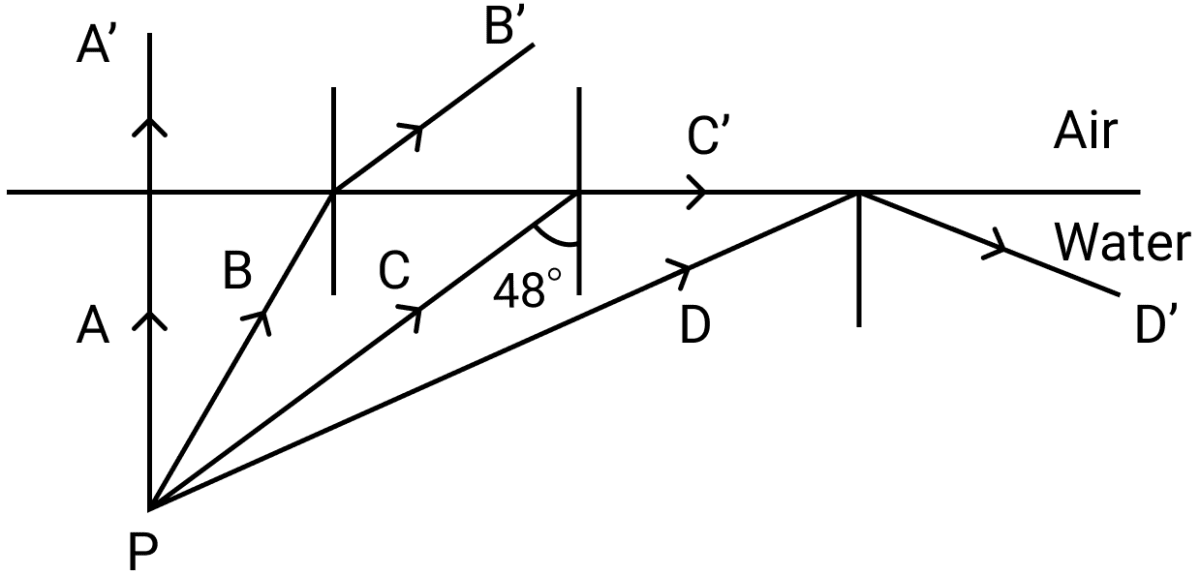(ii) The ray B exhibits refraction while the ray D exhibits total internal reflection.

#### Question 8(a)

Name the factor that determines: 

(i) Loudness of the sound heard.

(ii) Quality of the note.

(iii) Pitch of the note.

(i) The amplitude of wave determines the loudness of the sound heard.

(ii) The wave form determines the quality of the note.

(ii) The frequency of wave determines the pitch of the note.

#### Question 8(b)

(i) What are damped vibrations? 

(ii) Give one example of damped vibrations.

(iii) Name the phenomenon that causes a loud sound when the stem of a vibrating tuning fork is kept pressed on the surface of a table.

(i) The periodic vibrations of a body of decreasing amplitude in presence of a resistive force are called damped vibrations.

(ii) When a slim branch of a tree is pulled and then released, it makes the damped vibrations.

(iii) Resonance

#### Question 8(c)

(i) A wire of length 80 cm has a frequency of 256 Hz. Calculate the length of a similar wire under similar tension, which will have frequency 1024 Hz. 

(ii) A certain sound has a frequency of 256 hertz and a wavelength of 1.3 m.

1. Calculate the speed with which this sound travels.

2. What difference would be felt by a listener between the above sound and another sound travelling at the same speed, but of wavelength 2.6 m?

(i) Given,

l1 = 80 cm, f1 = 256 Hz, f2 = 1024 Hz, l2 = ?

Since, f ∝ $\dfrac{1}{l}$

Therefore, f1l1 = f2l2

or

$f_2 = \dfrac{f_1l_1}{f_2} \\[0.5em] = \dfrac{256 \times 80}{1024} \\[0.5em] = 20 \text{ cm}$

(ii) Given, f = 256 Hz, λ = 1.3 m

(a) V = fλ = 256 x 1.3 = 332.8 m s-1

(b) Speed of second sound (V') = 332.8 m s-1

Wavelength of second sound (λ') = 2.6 m

$\text{f} = \dfrac{V'}{λ'} \\[0.5em] = \dfrac{332.8}{2.6} \\[0.5em] = 128 \text{ Hz}$

As frequency of second sound is less as compared to the first sound, hence, to the listener, the first sound of wavelength 1.3 m will appear to be shriller than the second sound of wavelength 2.6 m.

#### Question 9(a)

(i) Name the colour code of the wire which is connected to the metallic body of an appliance. 

(ii) Draw the diagram of a dual control switch when the appliance is switched 'ON'.

(i) Green is the colour code of the wire which is connected to the metallic body of an appliance.

(ii) Below diagram shows the dual control switch in ON condition: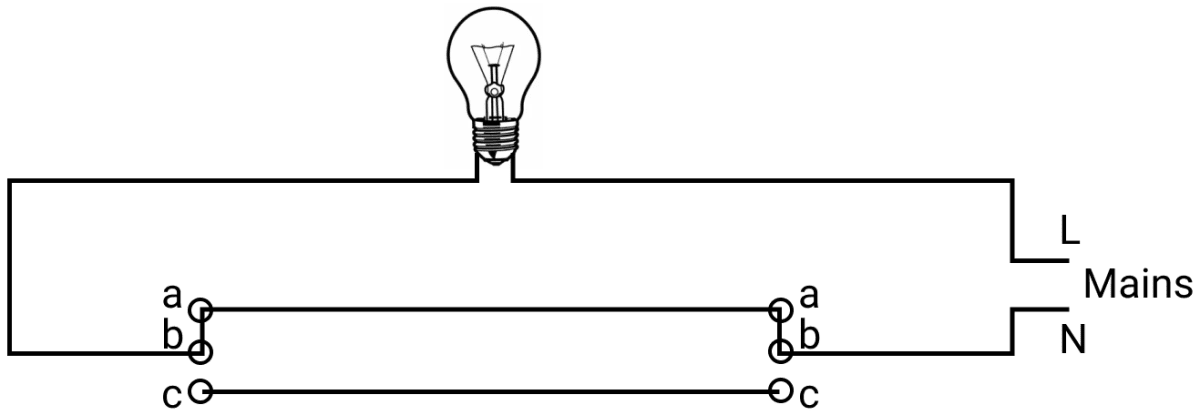#### Question 9(b)

(i) Which particles are responsible for current in conductors ? 

(ii) To which wire of a cable in a power circuit should the metal case of a geyser be connected?

(iii) To which wire should the fuse be connected?

(i) Free electrons are responsible for flow of current in conductors.

(ii) Earth wire (green) is connected to the metal case of a geyser.

(iii) The fuse is connected to the live wire (red or brown).

#### Question 9(c)

(i) Explain the meaning of the statement 'current rating of a fuse is 5A'. 

(ii) In the transmission of power, the voltage of power generated at the generating stations is stepped up from 11 kV to 132 kV before it is transmitted. Why?

(i) Current rating of a fuse is 5A, implies that if the current in a circuit having this fuse in the live wire exceeds 5A, it blows off and the circuit becomes incomplete without damaging the appliance connected in that circuit.

(ii) The power generated at the generating station is stepped up from 11 kV to 132 kV before transmission so as to reduce the magnitude of current to $\dfrac{1}{12}$th of it's value so that the heating loss of power in the line wires used for transmission are reduced [since heat produced ∝ (current)2]

#### Question 10(a)

Answer the following questions based on a hot cathode ray tube. 

(i) Name the charged particles.

(ii) State the approximate voltage used to heat the filament.

(iii) What will happen to the beam when it passes through the electric field?

(i) Cathode ray (or electrons)

(ii) 6 volt.

(iii) The beam will deflect towards the positive plate.

#### Question 10(b)

State three factors on which the rate of emission of electrons from a metal surface depends. 

The three factors on which the rate of emission of electrons from a metal surface depends are :

1. Work function of the metal.
2. The temperature of metal surface.
3. The surface area of the metal.

#### Question 10(c)

(i) What are free electrons? 

(ii) Why do they not leave the metal surface on their own?

(iii) How can they be made to leave the metal surface? (State any two ways)